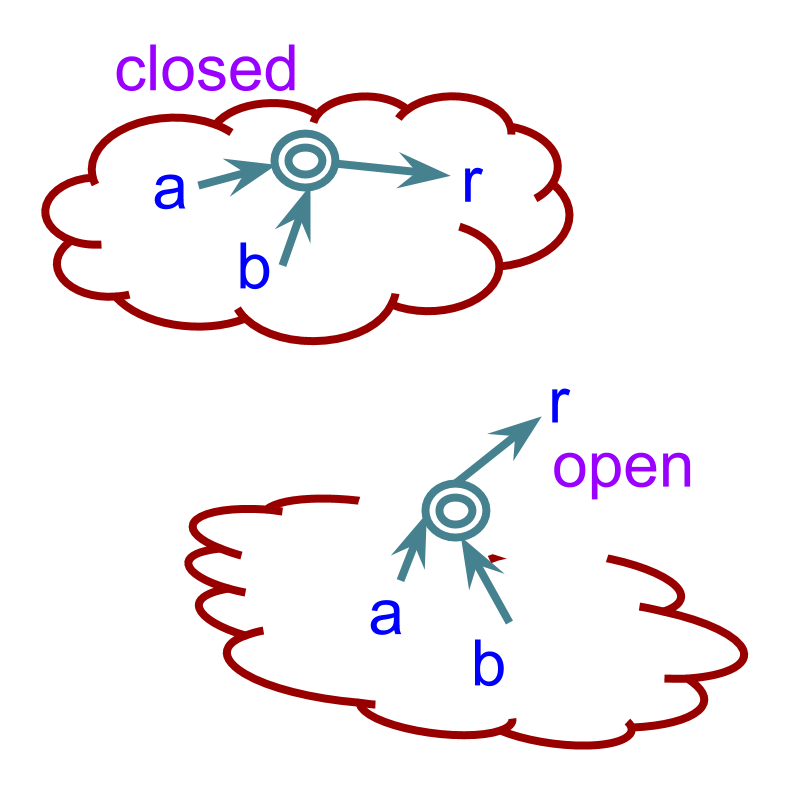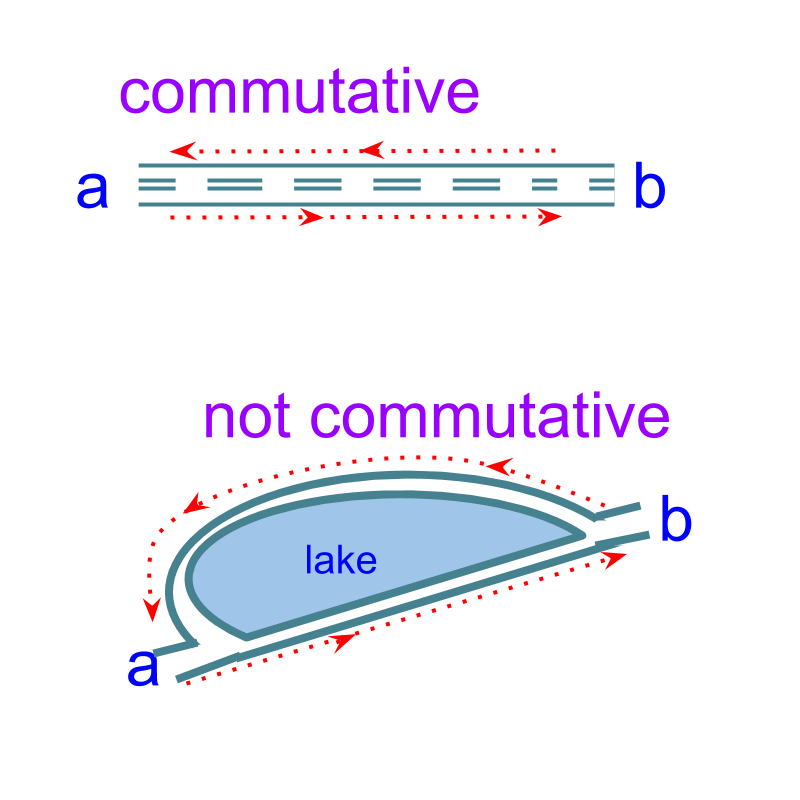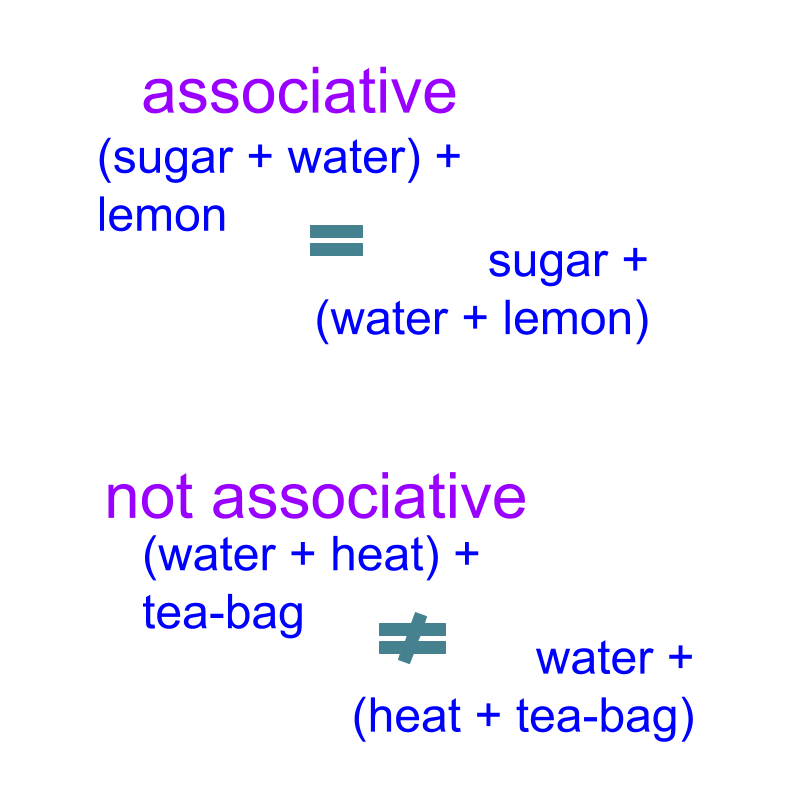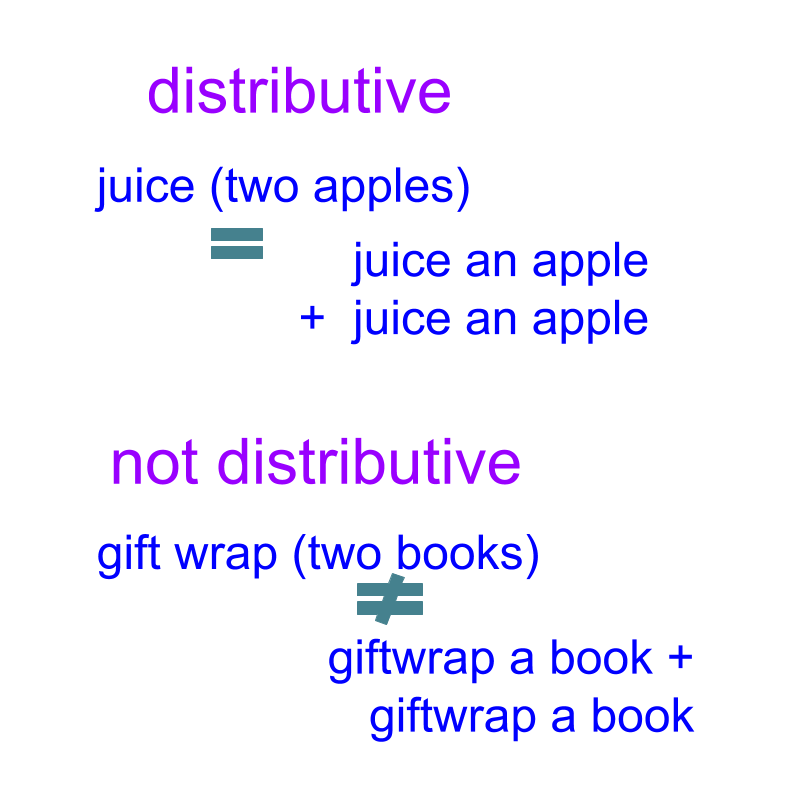maths > complex-number

Properties of Complex Multiplication

what you'll learn...

Overview

»  Complex Multiplication is closed.
→  ${z}_{1}×{z}_{2}\in ℂ$${z}_{1} \times {z}_{2} \in \mathbb{C}$

»  Complex Multiplication is commutative.
→  ${z}_{1}×{z}_{2}={z}_{2}×{z}_{1}$${z}_{1} \times {z}_{2} = {z}_{2} \times {z}_{1}$

»  Complex Multiplication is associative.
→  ${z}_{1}×\left({z}_{2}×{z}_{3}\right)=\left({z}_{1}×{z}_{2}\right)×{z}_{3}$${z}_{1} \times \left({z}_{2} \times {z}_{3}\right) = \left({z}_{1} \times {z}_{2}\right) \times {z}_{3}$

»  Complex Multiplicative identity is $1$$1$.
→  ${z}_{1}×1={z}_{1}$${z}_{1} \times 1 = {z}_{1}$

»  Complex Multiplicative inverse of $z$$z$ is $\frac{1}{z}$$\frac{1}{z}$.
→  $z×\frac{1}{z}=1$$z \times \frac{1}{z} = 1$

»  Complex Multiplication is distributive over complex addition.
→  ${z}_{1}×\left({z}_{2}+{z}_{3}\right)={z}_{1}×{z}_{2}+{z}_{1}×{z}_{3}$${z}_{1} \times \left({z}_{2} + {z}_{3}\right) = {z}_{1} \times {z}_{2} + {z}_{1} \times {z}_{3}$

»  Modulus of product is product of modulus.
→  $|{z}_{1}×{z}_{2}|=|{z}_{1}|×|{z}_{2}|$$| {z}_{1} \times {z}_{2} | = | {z}_{1} | \times | {z}_{2} |$

»  argument of product is sum of arguments of multiplicand and multiplier.
→  $arg\left({z}_{1}×{z}_{2}\right)=arg\left({z}_{1}\right)+arg\left({z}_{2}\right)$$a r g \left({z}_{1} \times {z}_{2}\right) = a r g \left({z}_{1}\right) + a r g \left({z}_{2}\right)$

closure lawThe word 'closure' means, closed and not open

Given complex numbers ${z}_{1}={a}_{1}+{b}_{1}i$${z}_{1} = {a}_{1} + {b}_{1} i$ and ${z}_{2}={a}_{2}+{b}_{2}i$${z}_{2} = {a}_{2} + {b}_{2} i$, ${a}_{1},{a}_{2},{b}_{1},{b}_{2}\in R$${a}_{1} , {a}_{2} , {b}_{1} , {b}_{2} \in R$,

${z}_{1}×{z}_{2}={a}_{1}{a}_{2}-{b}_{1}{b}_{2}+i\left({a}_{1}{b}_{2}+{a}_{2}{b}_{1}\right)$${z}_{1} \times {z}_{2} = {a}_{1} {a}_{2} - {b}_{1} {b}_{2} + i \left({a}_{1} {b}_{2} + {a}_{2} {b}_{1}\right)$,

It is noted that ${a}_{1}{a}_{2}-{b}_{1}{b}_{2}$${a}_{1} {a}_{2} - {b}_{1} {b}_{2}$ and ${a}_{1}{b}_{2}+{a}_{2}{b}_{1}$${a}_{1} {b}_{2} + {a}_{2} {b}_{1}$ are real numbers (by closure law of real-number addition, subtraction, and multiplication) and so, ${z}_{1}×{z}_{2}$${z}_{1} \times {z}_{2}$is a complex number.

Consider ${z}_{1}÷{z}_{2}$${z}_{1} \div {z}_{2}$

The complex number division is equivalently multiplication by the conjugate. So, ${z}_{1}÷{z}_{2}={z}_{1}×\overline{{z}_{2}}/{|{z}_{2}|}^{2}$${z}_{1} \div {z}_{2} = {z}_{1} \times \overline{{z}_{2}} / | {z}_{2} {|}^{2}$ is a complex number

summary

Closure property of Multiplication and Division: For any given complex numbers ${z}_{1},{z}_{2}\in ℂ$${z}_{1} , {z}_{2} \in \mathbb{C}$, ${z}_{1}\frac{×}{÷}{z}_{2}\in ℂ$${z}_{1} \frac{\times}{\div} {z}_{2} \in \mathbb{C}$.

Product or quotient of two complex number is another complex number - closed

Commutative LawThe word 'commute' means 'to go to and fro between two places on a regular basis'.

Consider the multiplication and division of complex numbers. ${z}_{3}={z}_{1}×{z}_{2}$${z}_{3} = {z}_{1} \times {z}_{2}$ given as ${a}_{3}+i{b}_{3}=\left({a}_{1}{a}_{2}-{b}_{1}{b}_{2}\right)+i\left({a}_{2}{b}_{1}+{a}_{1}{b}_{2}\right)$${a}_{3} + i {b}_{3} = \left({a}_{1} {a}_{2} - {b}_{1} {b}_{2}\right) + i \left({a}_{2} {b}_{1} + {a}_{1} {b}_{2}\right)$, where ${a}_{1},{a}_{2},{b}_{1},{b}_{2}\in ℝ$${a}_{1} , {a}_{2} , {b}_{1} , {b}_{2} \in \mathbb{R}$.
${z}_{4}={z}_{2}×{z}_{1}$${z}_{4} = {z}_{2} \times {z}_{1}$ $=\left({a}_{2}{a}_{1}-{b}_{2}{b}_{1}\right)+i\left({a}_{1}{b}_{2}+{a}_{2}{b}_{1}\right)$$= \left({a}_{2} {a}_{1} - {b}_{2} {b}_{1}\right) + i \left({a}_{1} {b}_{2} + {a}_{2} {b}_{1}\right)$

It is noted that ${z}_{3}={z}_{4}$${z}_{3} = {z}_{4}$ as the real and imaginary parts of the two complex numbers are equal by commutative law of real number multiplication.

${z}_{1}×{z}_{2}={z}_{2}×{z}_{1}$${z}_{1} \times {z}_{2} = {z}_{2} \times {z}_{1}$ will have the same

Commutative property is not defined for inverse operations. Division is the inverse of multiplication.

Division is the inverse of multiplication.

The commutative property has to be used in the following way.
${z}_{1}÷{z}_{2}$${z}_{1} \div {z}_{2}$
$\quad \quad = {z}_{1} \times \left(\frac{1}{z} _ 2\right)$
$\quad \quad = \left(\frac{1}{z} _ 2\right) \times {z}_{1}$ (commutative property of multiplication)

Given for two complex numbers ${z}_{1},{z}_{2}$${z}_{1} , {z}_{2}$; ${z}_{1}×{z}_{2}=3.1+i2.9$${z}_{1} \times {z}_{2} = 3.1 + i 2.9$. Find the value of ${z}_{2}×{z}_{1}$${z}_{2} \times {z}_{1}$.

The answer is '$3.1+i2.9$$3.1 + i 2.9$'

summary

Commutative Property of Complex Multiplication: for any complex number ${z}_{1},{z}_{2}\in ℂ$${z}_{1} , {z}_{2} \in \mathbb{C}$
${z}_{1}×{z}_{2}={z}_{2}×{z}_{1}$${z}_{1} \times {z}_{2} = {z}_{2} \times {z}_{1}$

Complex numbers can be swapped in complex multiplication - commutative.

associative lawThe word 'Associate' means 'to connect with; to join'.

When three complex numbers are multiplies,
•  Person A associates the middle complex number with first complex number and, to the result of that, he multiplies the third complex number $\left({z}_{1}×{z}_{2}\right)×{z}_{3}$$\left({z}_{1} \times {z}_{2}\right) \times {z}_{3}$
•  Person B associates the middle complex number with third complex number and to that she multiplies the first complex number ${z}_{1}×\left({z}_{2}×{z}_{3}\right)$${z}_{1} \times \left({z}_{2} \times {z}_{3}\right)$.

We can work out this in terms of real and imaginary parts of each number. It will prove that $\left({z}_{1}×{z}_{2}\right)×{z}_{3}$$\left({z}_{1} \times {z}_{2}\right) \times {z}_{3}$ $={z}_{1}×\left({z}_{2}×{z}_{3}\right)$$= {z}_{1} \times \left({z}_{2} \times {z}_{3}\right)$

Associative property is not applicable to division. Instead, Division is handled as inverse of multiplication and assiciative property of multiplication is applicable. i.e. For $\left({z}_{1}÷{z}_{2}\right)÷{z}_{3}$$\left({z}_{1} \div {z}_{2}\right) \div {z}_{3}$, it will be handled $\left({z}_{1}×\frac{1}{{z}_{2}}\right)×\frac{1}{{z}_{3}}$$\left({z}_{1} \times \frac{1}{z} _ 2\right) \times \frac{1}{z} _ 3$, then associative property of multiplication applies.

Given ${z}_{1},{z}_{2}\in ℂ$${z}_{1} , {z}_{2} \in \mathbb{C}$, what will be the result of ${z}_{1}×\left({z}_{2}×{z}_{1}\right)$${z}_{1} \times \left({z}_{2} \times {z}_{1}\right)$?

The answer is '${z}_{1}^{2}×{z}_{2}$${z}_{1}^{2} \times {z}_{2}$'.

summary

Associative property of Complex Multiplication: For Any complex number ${z}_{1},{z}_{2},{z}_{3}\in ℂ$${z}_{1} , {z}_{2} , {z}_{3} \in \mathbb{C}$,
${z}_{1}×\left({z}_{2}×{z}_{3}\right)=\left({z}_{1}×{z}_{2}\right)×{z}_{3}$${z}_{1} \times \left({z}_{2} \times {z}_{3}\right) = \left({z}_{1} \times {z}_{2}\right) \times {z}_{3}$

Order of multiplication can be changed - associative

multiplicative identity

The word "identical" means "exactly alike; same in every detail"

Consider the complex number $1+i0$$1 + i 0$. The result of multiplying that with a complex number ${z}_{1}={a}_{1}+i{b}_{1}$${z}_{1} = {a}_{1} + i {b}_{1}$ is '${z}_{1}$${z}_{1}$'.

The product is identical to the number being multiplied.

Multiplicative identity is 'an element, when multiplied, will result in product identical to the multiplicand'.

Consider ${z}_{1}=\sqrt{2}+\sqrt{3}i$${z}_{1} = \sqrt{2} + \sqrt{3} i$ What will be the product ${z}_{1}×\left(1+i0\right)$${z}_{1} \times \left(1 + i 0\right)$?

The answer is '${z}_{1}$${z}_{1}$'

summary

Multiplicative Identity in Complex Numbers: For any complex number ${z}_{1}\in ℂ$${z}_{1} \in \mathbb{C}$, there exists $1=1+i0,\in ℂ$$1 = 1 + i 0 , \in \mathbb{C}$ such that
${z}_{1}×1={z}_{1}$${z}_{1} \times 1 = {z}_{1}$

$1=1+i0$$1 = 1 + i 0$ is the multiplicative identity.

multiplicative inverse

The word 'inverse' means 'opposite; converse'.

Consider two complex numbers ${z}_{1}=a+ib$${z}_{1} = a + i b$ and ${z}_{2}=\frac{1}{a+ib}$${z}_{2} = \frac{1}{a + i b}$

${z}_{1}×{z}_{2}$${z}_{1} \times {z}_{2}$ is $1$$1$ multiplicative identity.

${z}_{2}=\frac{1}{a+ib}$${z}_{2} = \frac{1}{a + i b}$
$\quad \quad \quad = \frac{1}{a + i b} \times \frac{a - i b}{a - i b}$
$\quad \quad \quad = \frac{a - i b}{{a}^{2} + {b}^{2}}$

$\frac{1}{z}$$\frac{1}{z}$ or (a-ib)/(a^2+b^2) is the multiplicative inverse.

What is the multiplicative inverse of $2-i$$2 - i$?

The answer is '$\frac{2+i}{5}$$\frac{2 + i}{5}$'

summary

Multiplicative Inverse: For any complex number $z=a+ib\in ℂ$$z = a + i b \in \mathbb{C}$, there exists $\frac{1}{z}=\frac{a-ib}{{a}^{2}+{b}^{2}}\in ℂ$$\frac{1}{z} = \frac{a - i b}{{a}^{2} + {b}^{2}} \in \mathbb{C}$ such that
$z×\frac{1}{z}=1$$z \times \frac{1}{z} = 1$

For any complex number $z$$z$ there exists $\frac{1}{z}$$\frac{1}{z}$ : multiplicative inverse

distributive propertyThe word 'distribute' means 'to share; to spread'

Consider three complex numbers ${z}_{1},{z}_{2},{z}_{3}\in ℂ$${z}_{1} , {z}_{2} , {z}_{3} \in \mathbb{C}$, and the following

add first ${z}_{2}+{z}_{3}$${z}_{2} + {z}_{3}$ and multiply that by ${z}_{1}$${z}_{1}$. That is, ${z}_{1}×\left({z}_{2}+{z}_{3}\right)$${z}_{1} \times \left({z}_{2} + {z}_{3}\right)$
multiply ${z}_{1}×{z}_{2}$${z}_{1} \times {z}_{2}$ and ${z}_{1}×{z}_{3}$${z}_{1} \times {z}_{3}$ and added the results. That is ${z}_{1}×{z}_{2}+{z}_{1}×{z}_{3}$${z}_{1} \times {z}_{2} + {z}_{1} \times {z}_{3}$
These two results will be the same".

The complex numbers addition and multiplication is considered to be operations on numerical expressions of real numbers.

${z}_{1}×\left({z}_{2}+{z}_{3}\right)$${z}_{1} \times \left({z}_{2} + {z}_{3}\right)$
$\quad \quad = {z}_{1} \times {z}_{2} + {z}_{1} \times {z}_{3}$

proof:
${z}_{1}×\left({z}_{2}+{z}_{3}\right)$${z}_{1} \times \left({z}_{2} + {z}_{3}\right)$
$\quad \quad = \left({a}_{1} + i {b}_{1}\right) \times \left({a}_{2} + {a}_{3} + i \left({b}_{2} + {b}_{3}\right)\right)$
$\quad \quad = {a}_{1} {a}_{2} - {b}_{1} {b}_{2} + i \left({a}_{1} {b}_{2} + {a}_{2} {b}_{1}\right)$
$\quad \quad \quad \quad + {a}_{1} {a}_{3} - {b}_{1} {b}_{3} + i \left({a}_{1} {b}_{3} + {a}_{3} {b}_{1}\right)$
$\quad \quad = {z}_{1} \times {z}_{2} + {z}_{1} \times {z}_{3}$

Given ${z}_{1}{z}_{2}=3+4i$${z}_{1} {z}_{2} = 3 + 4 i$ and ${z}_{2}{z}_{3}=1-3i$${z}_{2} {z}_{3} = 1 - 3 i$ what is $\left({z}_{1}+{z}_{3}\right){z}_{2}$$\left({z}_{1} + {z}_{3}\right) {z}_{2}$?

The answer is '$4+i$$4 + i$'

summary

Distributive Property of Complex Numbers: For any given complex numbers ${z}_{1},{z}_{2},{z}_{3}\in ℂ$${z}_{1} , {z}_{2} , {z}_{3} \in \mathbb{C}$
${z}_{1}×\left({z}_{2}+{z}_{3}\right)$${z}_{1} \times \left({z}_{2} + {z}_{3}\right)$
$\quad \quad = {z}_{1} \times {z}_{2} + {z}_{1} \times {z}_{3}$

Product with sum of complex numbers is sum of, products with the complex numbers - distributive.

modulus in multiplication

Given ${z}_{1}={a}_{1}+i{b}_{1}$${z}_{1} = {a}_{1} + i {b}_{1}$ and ${z}_{2}={a}_{2}+i{b}_{2}$${z}_{2} = {a}_{2} + i {b}_{2}$. The modulus of product $|{z}_{1}×{z}_{2}|$$| {z}_{1} \times {z}_{2} |$ is '$|{z}_{1}|×|{z}_{2}|$$| {z}_{1} | \times | {z}_{2} |$'.

Given ${z}_{1}={a}_{1}+i{b}_{1}$${z}_{1} = {a}_{1} + i {b}_{1}$ and ${z}_{2}={a}_{2}+i{b}_{2}$${z}_{2} = {a}_{2} + i {b}_{2}$, the modulus of product
$|{z}_{1}×{z}_{2}|$$| {z}_{1} \times {z}_{2} |$
$\quad \quad = | {a}_{1} {a}_{2} - {b}_{1} {b}_{2} + i \left({b}_{1} {a}_{2} + {a}_{1} {b}_{2}\right) |$
$\quad \quad = \sqrt{{a}_{1}^{2} + {b}_{1}^{2}} \sqrt{{a}_{2}^{2} + {b}_{2}^{2}}$
$\quad \quad = | {z}_{1} | \times | {z}_{2} |$

Given ${z}_{1}={a}_{1}+i{b}_{1}$${z}_{1} = {a}_{1} + i {b}_{1}$ and ${z}_{2}={a}_{2}+i{b}_{2}$${z}_{2} = {a}_{2} + i {b}_{2}$ what is the modulus of quotient $|{z}_{1}÷{z}_{2}|$$| {z}_{1} \div {z}_{2} |$?

The answer is '$|{z}_{1}|÷|{z}_{2}|$$| {z}_{1} | \div | {z}_{2} |$'.

summary

Modulus of Product: For complex numbers ${z}_{1},{z}_{2}\in ℂ$${z}_{1} , {z}_{2} \in \mathbb{C}$

$|{z}_{1}×{z}_{2}|=|{z}_{1}|×|{z}_{2}|$$| {z}_{1} \times {z}_{2} | = | {z}_{1} | \times | {z}_{2} |$

Modulus of product is product of modulus.

argument in multiplication

Given ${z}_{1}={a}_{1}+i{b}_{1}$${z}_{1} = {a}_{1} + i {b}_{1}$ and ${z}_{2}={a}_{2}+i{b}_{2}$${z}_{2} = {a}_{2} + i {b}_{2}$, the argument of product $\text{arg}\left({z}_{1}×{z}_{2}\right)$$\textrm{a r g} \left({z}_{1} \times {z}_{2}\right)$ is 'text(arg)z_1 + text(arg)z_2'.

$\text{arg}\left({z}_{1}×{z}_{2}\right)$$\textrm{a r g} \left({z}_{1} \times {z}_{2}\right)$
$\quad \quad = \textrm{a r g} \left({r}_{1} {r}_{2} {e}^{i {\theta}_{1} + {\theta}_{2}}\right)$
$\quad \quad = {\theta}_{1} + {\theta}_{2}$
$\quad \quad = \textrm{a r g} {z}_{1} + \textrm{a r g} {z}_{2}$

Given ${z}_{1}={a}_{1}+i{b}_{1}$${z}_{1} = {a}_{1} + i {b}_{1}$ and ${z}_{2}={a}_{2}+i{b}_{2}$${z}_{2} = {a}_{2} + i {b}_{2}$, what is the argument of quotient $\text{arg}\left({z}_{1}÷{z}_{2}\right)$$\textrm{a r g} \left({z}_{1} \div {z}_{2}\right)$?

The answer is '$\text{arg}{z}_{1}-\text{arg}{z}_{2}$$\textrm{a r g} {z}_{1} - \textrm{a r g} {z}_{2}$'.

summary

Argument of Product: For complex numbers ${z}_{1},{z}_{2}\in ℂ$${z}_{1} , {z}_{2} \in \mathbb{C}$
$\text{arg}\left({z}_{1}×{z}_{2}\right)=\text{arg}{z}_{1}+\text{arg}{z}_{2}$$\textrm{a r g} \left({z}_{1} \times {z}_{2}\right) = \textrm{a r g} {z}_{1} + \textrm{a r g} {z}_{2}$

argument of product is sum of arguments.

Outline